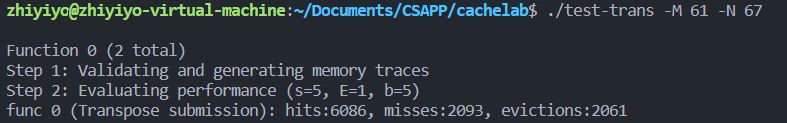# CSAPP 之 CacheLab 详解

2022-05-18 15:29 2819 查看

# 解题过程

## Part A

### 题目要求

Part A 给出了一些后缀名为

trace

[空格] 操作 地址,数据大小
，其中操作的类型有以下几种：

• I：取指令操作
• L：读数据操作
• S：写数据操作
• M：修改数据操作，比如先读一次数据再写一次数据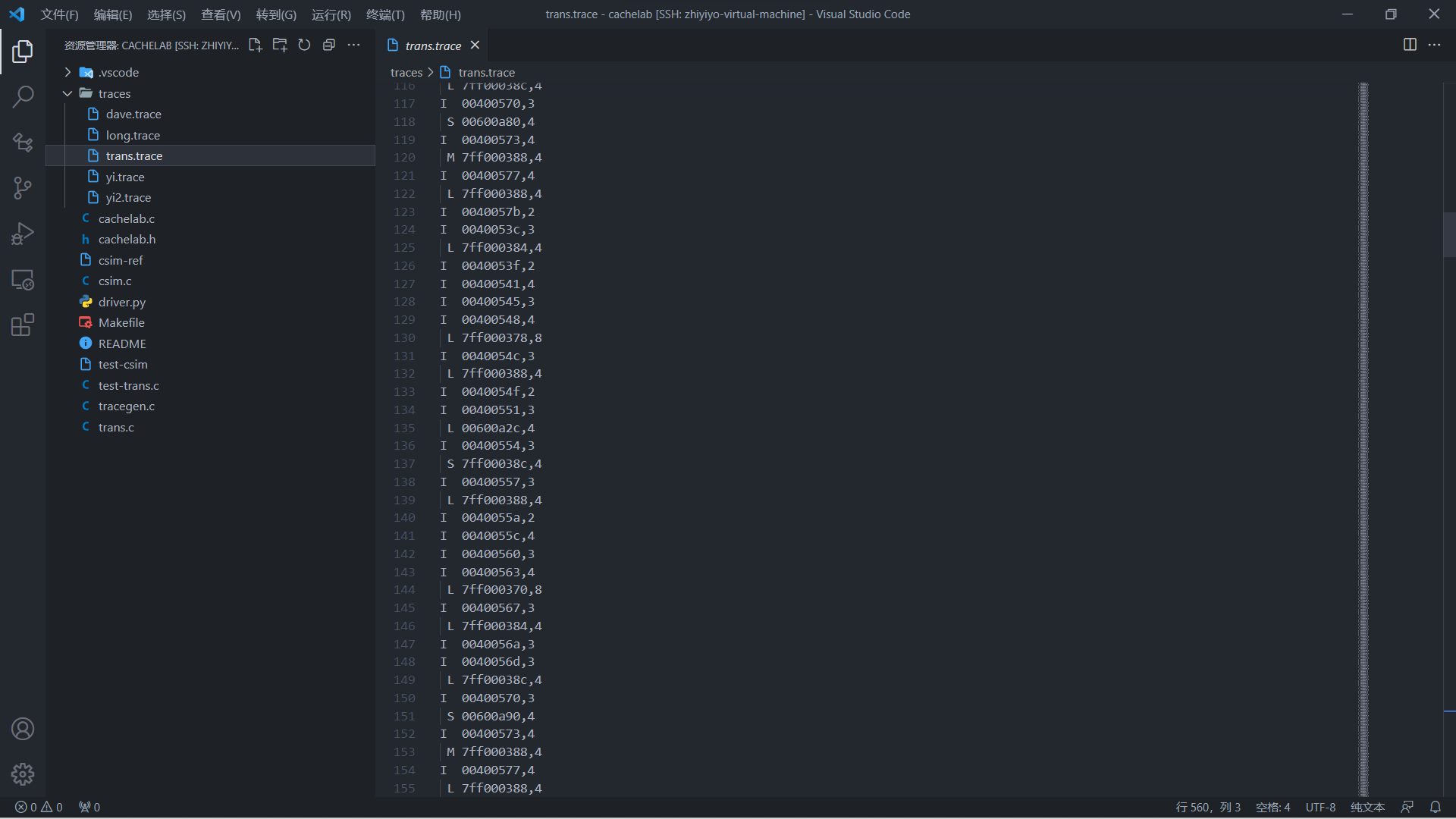Part A 要求实现的缓存存储器的行为和

csim-ref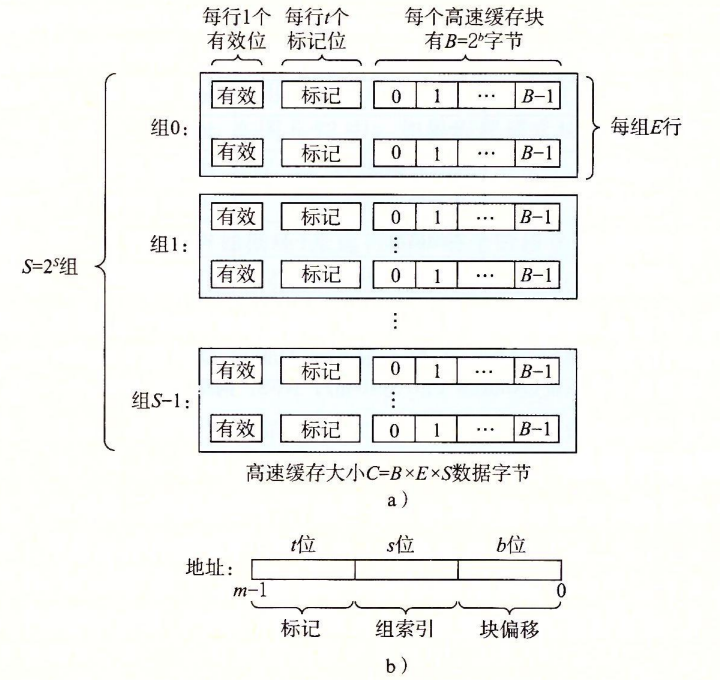• -s
：组索引的位数
• -E
：行数
• -b
：块大小 B=2^b 中的 b
• -t
trace
文件的路径

trace

csim

csim-ref

### 代码

time

typedef struct {
int valid;
int tag;
int time;
} CacheLine, *CacheSet, **Cache;

#include <assert.h>
#include <getopt.h>
#include <stdint.h>
#include <stdio.h>
#include <stdlib.h>
#include <string.h>
#include "cachelab.h"

int hit, miss, evict;

int s, S, E, b;
char filePath;

Cache cache;

int main(int argc, char* argv[]) {
int opt;
while ((opt = getopt(argc, argv, "s:E:b:t:")) != -1) {
switch (opt) {
case 's':
s = atoi(optarg);
S = 1 << s;
break;
case 'E':
E = atoi(optarg);
break;
case 'b':
b = atoi(optarg);
break;
case 't':
strcpy(filePath, optarg);
break;
}
}

mallocCache();
simulate();
freeCache();

printSummary(hit, miss, evict);
return 0;
}

malloc

/* 动态分配缓存空间 */
void mallocCache() {
cache = (Cache)malloc(S * sizeof(CacheSet));
assert(cache);

for (int i = 0; i < S; ++i) {
cache[i] = (CacheSet)malloc(E * sizeof(CacheLine));
assert(cache[i]);
}
}

/* 释放缓存空间 */
void freeCache() {
for (int i = 0; i < S; ++i) {
free(cache[i]);
}
free(cache);
}

trace

/* 模拟缓存读写操作*/
void simulate() {
FILE* file = fopen(filePath, "r");
assert(file);

char op;
int size;
while (fscanf(file, " %c %lx,%d", &op, &address, &size) > 0) {
switch (op) {
case 'M':
case 'L':
case 'S':
break;
}
lruUpdate();
}

fclose(file);
}

/* 更新访问时间 */
void lruUpdate() {
for (int i = 0; i < S; ++i) {
for (int j = 0; j < E; ++j) {
if (cache[i][j].valid) {
cache[i][j].time++;
}
}
}
}

tag

/* 访问缓存 */
int tag = address >> (b + s);
uint64_t mask = ((1ULL << 63) - 1) >> (63 - s);

// 缓存击中
for (int i = 0; i < E; ++i) {
if (cacheSet[i].valid && cacheSet[i].tag == tag) {
hit++;
cacheSet[i].time = 0;
return;
}
}

miss++;

// 有空位，直接写入
for (int i = 0; i < E; ++i) {
if (!cacheSet[i].valid) {
cacheSet[i].valid = 1;
cacheSet[i].tag = tag;
cacheSet[i].time = 0;
return;
}
}

// 没有空位，只能使用 LRU 算法进行替换
evict++;
int evictIndex = 0;
int maxTime = 0;
for (int i = 0; i < E; ++i) {
if (cacheSet[i].time > maxTime) {
maxTime = cacheSet[i].time;
evictIndex = i;
}
}

cacheSet[evictIndex].tag = tag;
cacheSet[evictIndex].time = 0;
}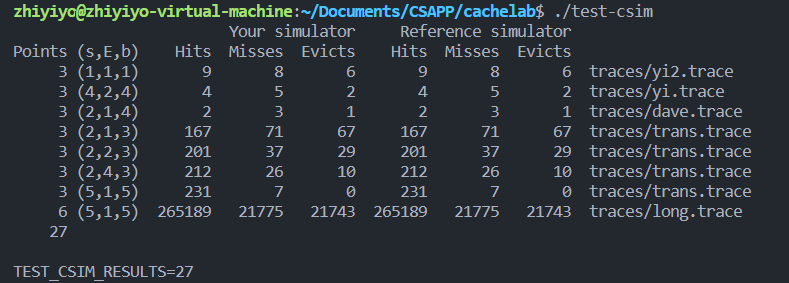## Part B

Part B 给出了最原始的转置操作代码：

void trans(int M, int N, int A
[M], int B[M]
) {
int i, j, tmp;

for (i = 0; i < N; i++) {
for (j = 0; j < M; j++) {
tmp = A[i][j];
B[j][i] = tmp;
}
}
}

• 使用分块技术进行优化
• 对角线上的元素会引发冲突未击中

for (int i = 0; i < N; i += 8)
for (int j = 0; j < M; j += 8)
for (int ii = i; ii < i + 8; ++ii)
for (int jj=j; jj < j + 8; ++jj)
B[jj][ii] = A[ii][jj];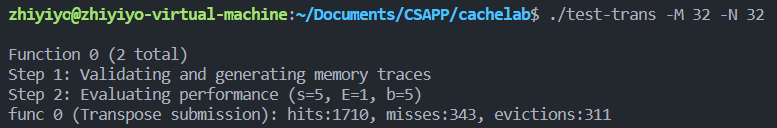int a, b, c, d, e, f, g, h;
for (int i = 0; i < N; i += 8) {
for (int j = 0; j < M; j += 8) {
for (int ii = i; ii < i + 8; ++ii) {
a = A[ii][j];
b = A[ii][j + 1];
c = A[ii][j + 2];
d = A[ii][j + 3];
e = A[ii][j + 4];
f = A[ii][j + 5];
g = A[ii][j + 6];
h = A[ii][j + 7];

B[j][ii] = a;
B[j + 1][ii] = b;
B[j + 2][ii] = c;
B[j + 3][ii] = d;
B[j + 4][ii] = e;
B[j + 5][ii] = f;
B[j + 6][ii] = g;
B[j + 7][ii] = h;
}
}
}int a, b, c, d;
for (int i = 0; i < N; i += 4) {
for (int j = 0; j < M; j += 4) {
for (int ii = i; ii < i + 4; ++ii) {
a = A[ii][j];
b = A[ii][j + 1];
c = A[ii][j + 2];
d = A[ii][j + 3];

B[j][ii] = a;
B[j + 1][ii] = b;
B[j + 2][ii] = c;
B[j + 3][ii] = d;
}
}
}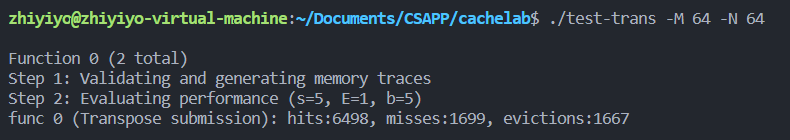int a, b, c, d, e, f, g, h;
int n = 8 * (N / 8);
int m = 8 * (M / 8);
for (int i = 0; i < n; i += 8) {
for (int j = 0; j < m; j += 8) {
for (int ii = i; ii < i + 8; ++ii) {
a = A[ii][j];
b = A[ii][j + 1];
c = A[ii][j + 2];
d = A[ii][j + 3];
e = A[ii][j + 4];
f = A[ii][j + 5];
g = A[ii][j + 6];
h = A[ii][j + 7];

B[j][ii] = a;
B[j + 1][ii] = b;
B[j + 2][ii] = c;
B[j + 3][ii] = d;
B[j + 4][ii] = e;
B[j + 5][ii] = f;
B[j + 6][ii] = g;
B[j + 7][ii] = h;
}
}
}

// 处理剩余部分
for (int i = 0; i < n; i++) {
for (int j = m; j < M; j++) {
B[j][i] = A[i][j];
}
}

for (int i = n; i < N; i++) {
for (int j = 0; j < M; j++) {
B[j][i] = A[i][j];
}
}